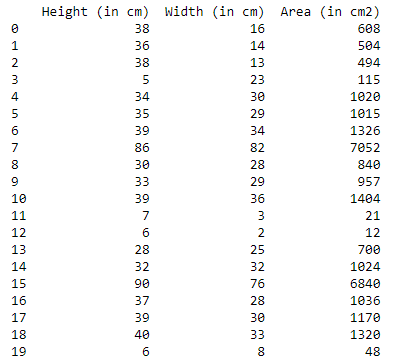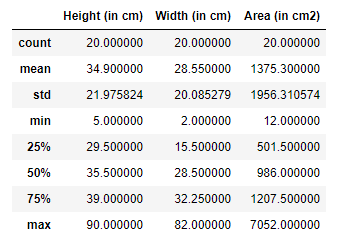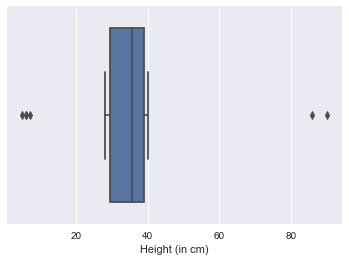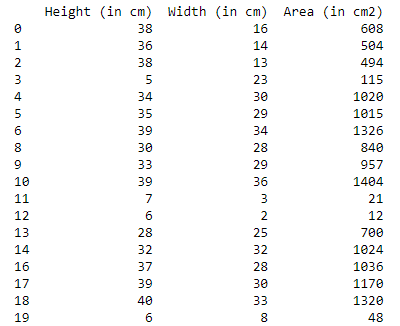# How to use Pandas filter with IQR?

• Last Updated : 02 Dec, 2021

The IQR or Inter Quartile Range is a statistical measure used to measure the variability in a given data. In naive terms, it tells us inside what range the bulk of our data lies. It can be calculated by taking the difference between the third quartile and the first quartile within a dataset.

`IQR = Q3 - Q1`

Where, Q3 = the 75th percentile value (it is the middle value between the median and the largest value inside a dataset). Q1 = the 25th percentile value (it is the middle value between the median and the smallest value inside a dataset). Also, Q2 denotes the 50th percentile i.e., the median of a dataset. For more information about IQR please read https://www.geeksforgeeks.org/interquartile-range-iqr/.

In this article, we will be knowing how to filter a dataset using Pandas with the help of IQR.

The Inter Quartile Range (IQR) is a methodology that is generally used to filter outliers in a dataset. Outliers are extreme values that lie far from the regular observations that can possibly be got generated because of variability in measurement or experimental error. Many a time we want to identify these outliers and filter them out to reduce errors. Here, we will be showing an example to detect outliers and filter them out using Pandas in Python programming language.

Let’s first begin by importing important libraries that we will require to identify and filter the outliers.

## Python

 `# Importing important libraries``import` `numpy as np``import` `pandas as pd``import` `seaborn as sns``import` `matplotlib.pyplot as plt``plt.style.use(``'seaborn'``)`

Now, we will read the dataset in which we want to detect and filter outliers. The dataset can be downloaded from https://tinyurl.com/gfgdata. It can be done using the read_csv() method present in the Pandas library and can be written as:

## Python

 `# Reading the dataset``data ``=` `pd.read_csv(``'Dataset.csv'``)``print``(``"The shape of the dataframe is: "``, data.shape)`

Output:

` The shape of the dataframe is:  (20, 4)`

Printing the dataset

We can print the dataset to have a look at the data.

## Python

 `print``(data)`

Our dataset looks like this:We can observe some statistical information about this dataset using data.describe() method, which can be done as:

## Python

 `data.describe()`

Output:It can be observed that features such as ‘Height’, ‘Width’, ‘Area’ have very deferred maximum value as compared to the 75% value, thus we can say there are certain observations that act as outliers in the dataset. Similarly, the minimum value in these columns differs greatly from the 25% value, so it signifies the presence of outliers.

It can be verified by plotting a box plot of the above features, here I’m plotting the box plot for the Height column and in the same manner box plot for other features can be plotted.

## Python

 `plt.figure(figsize``=``(``6``,``4``))``sns.boxplot(data[``'Height (in cm)'``])``plt.show()`

Output:We can observe the presence of outliers beyond the first quartile and the third quartile in the box plot.

To find out and filter such outliers in the dataset we will create a custom function that will help us remove outliers. In the function, we first need to find out the IQR value that can be calculated by finding the difference between the third and first quartile values. Secondly, we will write a query to select observations that lie outside the lower_range and upper_range IQR region and remove them. It can be written as:

## Python

 `# Removing the outliers``def` `removeOutliers(data, col):``    ``Q3 ``=` `np.quantile(data[col], ``0.75``)``    ``Q1 ``=` `np.quantile(data[col], ``0.25``)``    ``IQR ``=` `Q3 ``-` `Q1` `    ``print``(``"IQR value for column %s is: %s"` `%` `(col, IQR))``    ``global` `outlier_free_list``    ``global` `filtered_data` `    ``lower_range ``=` `Q1 ``-` `1.5` `*` `IQR``    ``upper_range ``=` `Q3 ``+` `1.5` `*` `IQR``    ``outlier_free_list ``=` `[x ``for` `x ``in` `data[col] ``if` `(``        ``(x > lower_range) & (x < upper_range))]``    ``filtered_data ``=` `data.loc[data[col].isin(outlier_free_list)]`  `for` `i ``in` `data.columns:``      ``if` `i ``=``=` `data.columns[``0``]:``      ``removeOutliers(data, i)``    ``else``:``      ``removeOutliers(filtered_data, i)` ` ` `# Assigning filtered data back to our original variable``data ``=` `filtered_data``print``(``"Shape of data after outlier removal is: "``, data.shape)`

Output:

```IQR value for column Height (in cm) is: 9.5
IQR value for column Width (in cm) is: 16.75
IQR value for column Area (in cm2) is: 706.0
Shape of data after outlier removal is:  (18, 3)```

Printing the data afterward we can notice two of our extreme observations which were acting as outliers get removed.

## Python

 `print``(data)`

Output:We can observe the rows with index numbers 7 and 15 got removed from the original dataset.

My Personal Notes arrow_drop_up